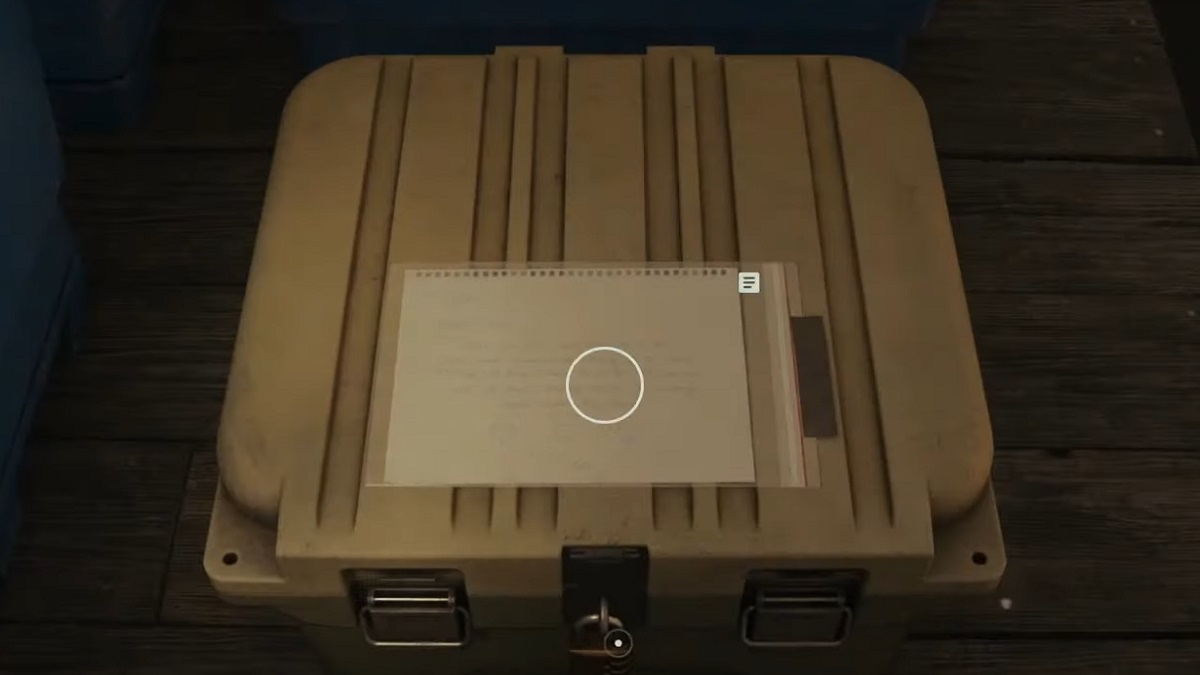# Alan Wake 2: How to Solve the Battery Cult Stash Puzzle

What's the answer to the 1600 Amp word problem in Alan Wake 2?Screenshot by GameSkinny

You’ll find Cult Stashes throughout the game, and each one has a puzzle that needs to be solved to open it. Here’s how to solve the Battery Cult Stash Puzzle in Alan Wake 2. The real trick to this Cult Stash is solving the math problem that’s required to open it.

## What’s the Answer to the Battery Cult Stash Puzzle in Alan Wake 2?

### Battery Stash Location

You’ll find this stash in Watery after the flood water goes down. It’s sitting on the easternmost dock among other boxes. On it is the puzzle, which reads:

There are three batteries (B1, B2, B3) which have a combined charge of 1600 Amps. B2 has 128 Amps more than B3. B1 has two times as much charge as B3. How many Amps does B2 have?

### Setting Up and Solving the Math Problem

I know that word problems aren’t generally beloved. However, I was a bit of a mathlete during my school years. This problem can be solved as a system of equations and we can rewrite each battery in terms of B3. This gives us:

• B2 = B3 + 128
• B1 = 2B3
• B1 + B2 + B3 = 1600

Then, we substitute B1 and B2 in the final equation with the equations that define them in terms of B3. This gives us a new equation that looks like:

• 2B3 + B3 + 128 + B3 = 1600

Afterwards, we solve it like this:

• 2B3 + 2B3 = 1472
• 4B3 = 1472
• B3 = 368

And then we substitute B3 back into our B2 = B3 + 128 equation to get B2 = 368 + 128 to reach the final answer of B2 = 496. This means that the code to unlock the stash is, from top to bottom, 4-9-6.

And that covers how to solve the Battery Cult Stash Puzzle in Alan Wake 2. In some cases, math is scarier than the enemies in a horror game, but luckily we’re here to help with guides. From here, check out our AW2 guide hub for less math-y content like how to open the Streamside Stash or how to find Witchfinder Station’s Computer Code.